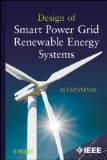Price : \$166.00

# Design of Smart Power Grid Renewable Energy Systems

To address the modeling and control of smart grid renewable energy system into electric power systems, this book integrates three areas of electrical engineering: power system engineering, control systems engineering and power electronics  The approach to the integration of these three areas differs from classical methods. Due to complexity of this task, the author has decided to present the basic concepts, and then present a simulation test bed in matlab to use these concepts to solve a basic problem in development of smart grid energy system. Therefore, each chapter has three parts: first a problem of integration is stated and its importance is described. Then, the mathematical model of the same problem is formulated. Next, the solution steps are outlined. This step is followed by developing a matlab simulation test bed. Each chapter ends with a set of problems and projects. The book is intended be used as textbook for instruction or by researchers.  This book can be used as undergraduate text for both electrical and mechanical engineers. The prerequisite for the course is a course in fundamental of electrical engineering.